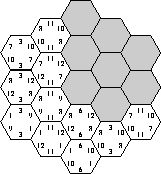시간 제한메모리 제한제출정답맞힌 사람정답 비율
1 초 128 MB0000.000%

## 문제

Consider a game board consisting of 19 hexagonal fields, as shown in the figure below. We can easily distinguish three main directions in the shape of the board: from top to bottom, from top-left to bottom-right, and from top-right to bottom-left. For each of these primary directions, the board can be viewed as a series of rows, consisting of 3, 4, 5, 4, and 3 fields, respectively.The game board has to be completely covered using a set of hexagonal pieces. Each piece carries three numbers, one for every primary board direction. Only three different numbers are used for each direction. Every possible combination of three numbers for all three directions is assigned to a piece, leading to a set of 27 unique pieces. (The board in the above figure is still in the process of being covered.)

The score of a board is calculated as the sum of all 15 row scores (5 rows for each primary direction). The row scores are calculated as follows: if all pieces in a row carry the same number for the direction of the row, the row score is this number multiplied by the number of pieces in the row. Otherwise (the pieces carry different numbers in the row direction) the row score is zero. Note that the pieces may not be rotated. For example, the score of the leftmost row in the figure is 3 . 3 = 9, the score of the row to its right is 4 . 11 = 44.

While in the real game the pieces are chosen randomly and the set of pieces is fixed, we are interested in the highest possible score for a given set of numbers for each direction. This means you have to choose those 19 pieces that result in the highest score.

## 입력

The first line of the input file contains an integer n which indicates the number of test cases. Each test case consists of three lines containing three integers each. Each of these three line contains the numbers for a single primary direction. From these numbers the set of pieces is generated.

## 출력

For each test case output a line containing the number of the case (Test #1', Test #2', etc.), followed by a line containing the highest possible score for the given numbers. Add a blank line after each test case.

## 예제 입력 1

1
9 4 3
8 5 2
7 6 1


## 예제 출력 1

Test #1
308